# Introduction

This document serves as the main overview of the ggforce package, and will try to explain the hows and whys of the different extension along with clear visual examples. It will try to link back to relevant academic articles describing the different visualization types in more detail - both for the benefit of the reader but also to give credit to the people who thought long and hard about how to best present your data.

## Geom versions

Some of the geom versions presented below, comes in two or more flavors, potentially suffixed with 0 or 2, such as for `geom_bezier` which also comes in the versions `geom_bezier0` and `geom_bezier2`. This pattern is mainly used in line drawings such as splines, arcs and bezier and has been adopted for edge drawing in the ggraph package as well. In all cases the base version (no suffix) has been implemented efficiently in C++ and produces a set of points along the line, that can be traced using a path. The benefit of this is that the detail level can be chosen, thus giving the user control over the rendering time. On top of that, an additional column is added to the data with the position along the path, which can be used to map e.g. an opacity gradient to. For the base version each line is encoded in one row using `x`, `y`, `xend`, and `yend` in the same manner as known as `geom_segment`. The same input format is used for the 0-version, but this version maps directly to native grid grobs. While there is seldom a performance reason to use the native grobs, these version do ensure that the path is always smooth (For the base versions this is dependent on the number of points calculated). The 0-versions does not allow for mapping of gradients to the path. The 2-version changes the input format into encoding the start and end points on different rows in the same manner as for `geom_path`. The benefit of this is that different aesthetic variables can be defined for the start and end, e.g. colour, and these versions will make sure to interpolate that aesthetic along the path so you can get e.g. smooth transition of size, colour, and opacity along a spline.

# Layers

This section shows the extensions to ggplot2’s geoms and stats. It rarely makes sense to talk about one and not the other, so they are grouped together here. Often the focus will be on the geoms, unless a new stat does not have an accompanying geom, in which case the stat will be discussed along with which geoms it should be used with.

## Arcs

Arcs are segments of a circle and defined by a centre point, a radius and a start and end angle. In ggforce arcs come in two flavors: arc and arc_bar, where the former draws an arc with a single line and the latter draws it as a polygon that can have a fill and outline. A wedge is a special case of arc_bar where the innermost radius is 0. The most well known use of arcs in plotting is with the much loathed pie chart (and its cousin the donut chart). The reason for all the hatred against pie charts are just and related to the fact that humans are much better at comparing heights than angles. Because of this a bar chart will always communicate your data better than a pie chart. Donut charts are a little better as the hole in the middle forces the eye to compare arc spans rather than angles, but it is still better to use a bar chart. Arcs, being a fundamental visual element, can be used for other things though, such as sunburst plots or annotating radial visualizations.

As pie charts are most well known, we’ll start by upsetting all visualization expert and produce one:

``````# We'll start by defining some dummy data
pie <- data.frame(
state = c('eaten', 'eaten but said you didn\'t', 'cat took it',
'for tonight', 'will decompose slowly'),
focus = c(0.2, 0, 0, 0, 0),
start = c(0, 1, 2, 3, 4),
end = c(1, 2, 3, 4, 2*pi),
amount = c(4,3, 1, 1.5, 6),
stringsAsFactors = FALSE
)

p <- ggplot() + theme_no_axes() + coord_fixed()

# For low level control you define the start and end angles yourself
p + geom_arc_bar(aes(x0 = 0, y0 = 0, r0 = 0, r = 1, start = start, end = end,
fill = state),
data = pie)``````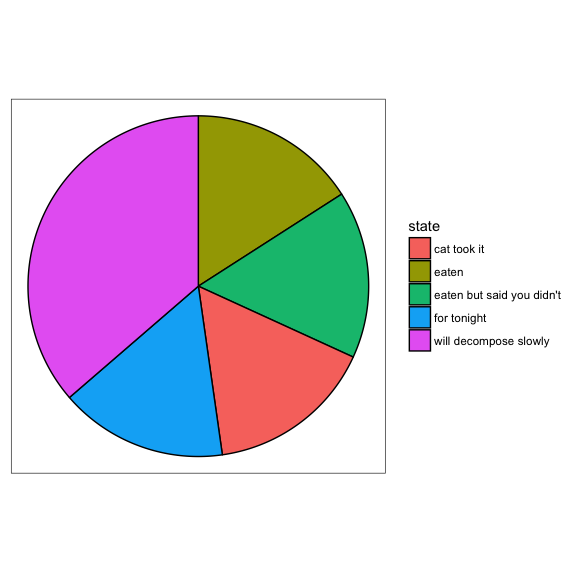``````# But often you'll have values associated with each wedge. Use stat_pie then
p + geom_arc_bar(aes(x0 = 0, y0 = 0, r0 = 0, r = 1, amount = amount,
fill = state),
data = pie, stat = 'pie')``````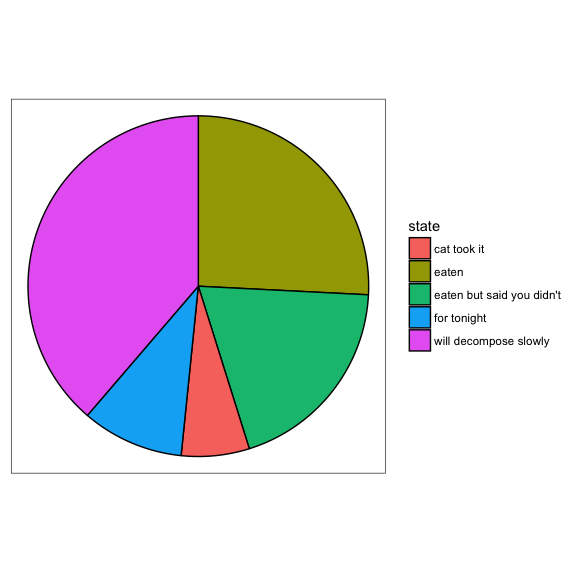``````# The wedges can be exploded away from the centre using the explode aesthetic
p + geom_arc_bar(aes(x0 = 0, y0 = 0, r0 = 0, r = 1, amount = amount,
fill = state, explode = focus),
data = pie, stat = 'pie')``````
``## Warning: Ignoring unknown aesthetics: explode``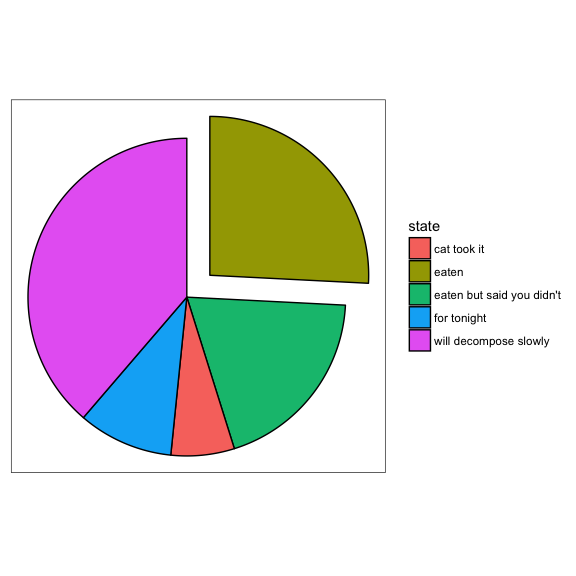``````# And a donut can be made by setting r0 to something > 0
p + geom_arc_bar(aes(x0 = 0, y0 = 0, r0 = 0.8, r = 1, amount = amount,
fill = state, explode = focus),
data = pie, stat = 'pie')``````
``## Warning: Ignoring unknown aesthetics: explode``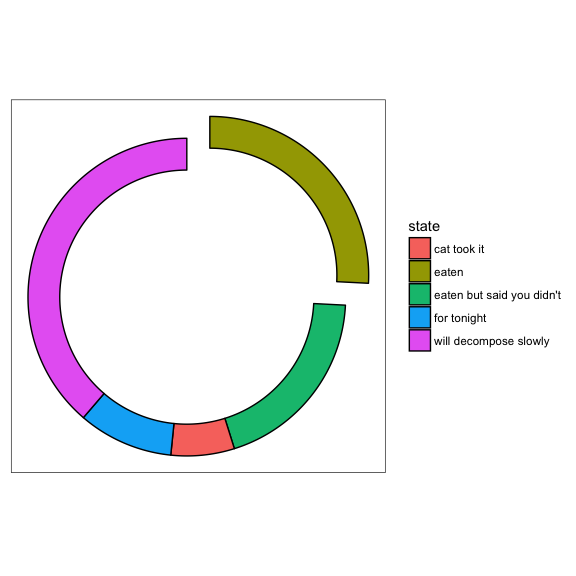While the above produces some of the most hated plot types in the world it does showcase the use of arcs in plotting. Arcs can be used in many different visualization types to annotate radial position etc. as in e.g. choord diagrams.

Using arc is just like arc_bar except that it does not take an r0 argument and does not have any fill. Furthermore the arc geoms contains the 0 and 2 versions making gradients and interpolation possible.

``````arcs <- data.frame(
start = 0,
end = runif(5) * 2*pi,
r = seq_len(5)
)
p <- ggplot() + theme_no_axes() + coord_fixed()

p + geom_arc(aes(x0 = 0, y0 = 0, r = r, start = start, end = end,
alpha = ..index.., colour = factor(r)), data = arcs)``````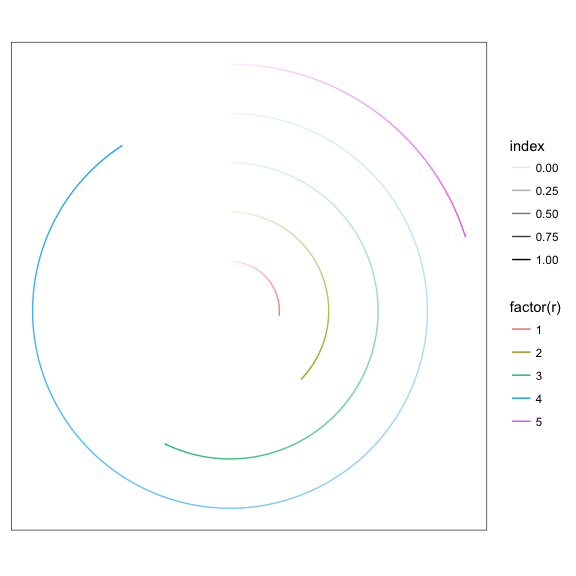``````# The 0 version will not properly expand the axes, as their extend is only
# known at draw time
p + geom_arc0(aes(x0 = 0, y0 = 0, r = r, start = start, end = end,
colour = factor(r)), data = arcs, ncp = 50)``````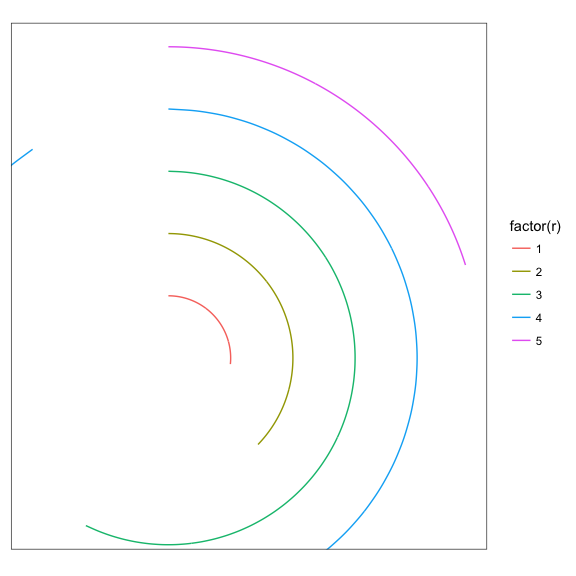``````# The 2 version allow you to create gradients, but the input data format is
# different
arcs <- rbind(data.frame(end = 0, r = 1:5), arcs[, c('end', 'r')])
arcs\$col <- sample(5, 10, TRUE)
p + geom_arc2(aes(x0 = 0, y0 = 0, r = r, group = r, end = end,
colour = factor(col)), data = arcs, size = 3)``````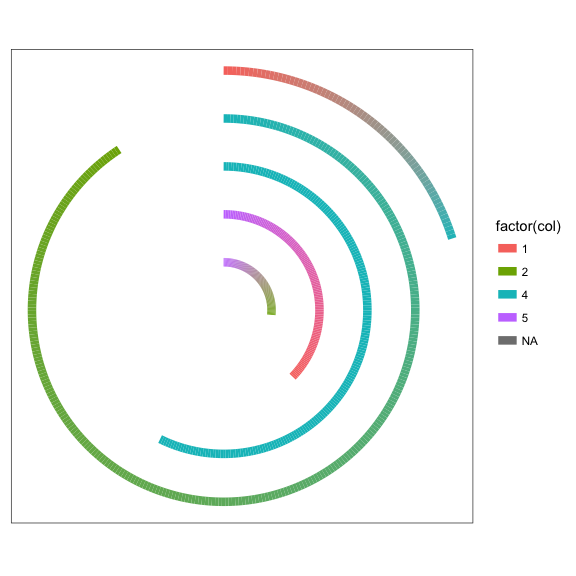## Circles

Standard ggplot2 generally has you covered when it comes to drawing circles through the point geom, it does not make it possible to draw circles where the radius of the circles are related to the coordinate system. The geom_circle from ggforce are precisely for that. It generates a polygon resembling a circle based on a center point and a radius, making the radius directly readable from the axes. The geom are mainly intended to make it possible to draw circles with fine grained control, but will often not have any utility in itself. An exception would be in plotting trees as enclosure diagrams using circles. Here it will be necessary to have fine control over radius.

``````# Here are some data describing some circles
circles <- data.frame(
x0 = rep(1:3, 2),
y0 =  rep(1:2, each=3),
r = seq(0.1, 1, length.out = 6)
)
ggplot() + geom_circle(aes(x0=x0, y0=y0, r=r, fill=r), data=circles)``````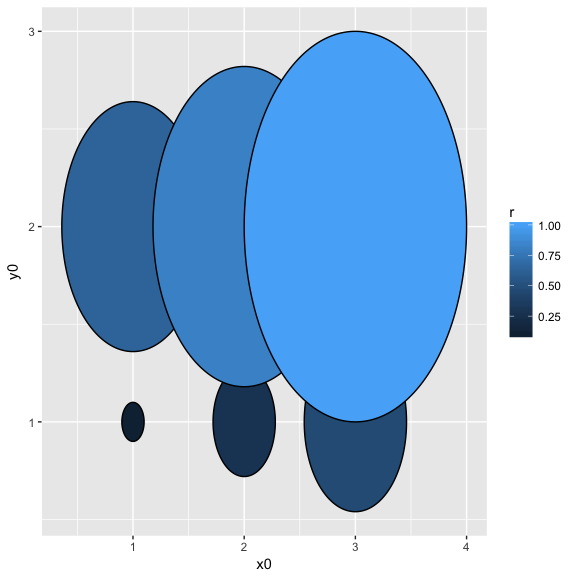``````# As it is related to the coordinate system, coord_fixed() is needed to ensure
# true circularity
ggplot() + geom_circle(aes(x0=x0, y0=y0, r=r, fill=r), data=circles) +
coord_fixed()``````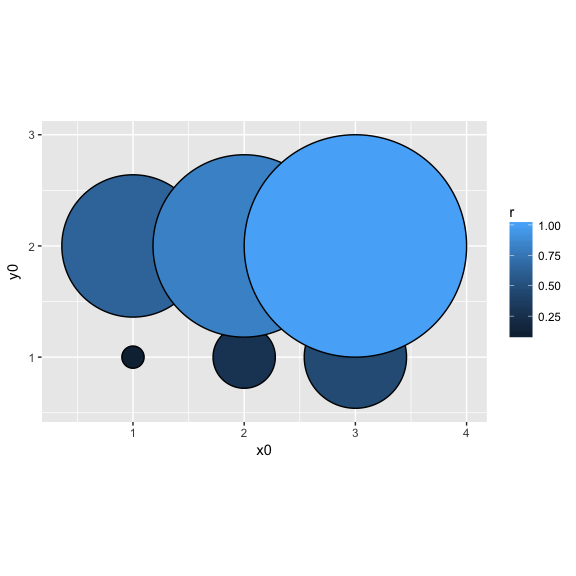``````# Use n to set the smoothness of the circle
ggplot() + geom_circle(aes(x0=x0, y0=y0, r=r, fill=r), data=circles, n=10) +
coord_fixed()``````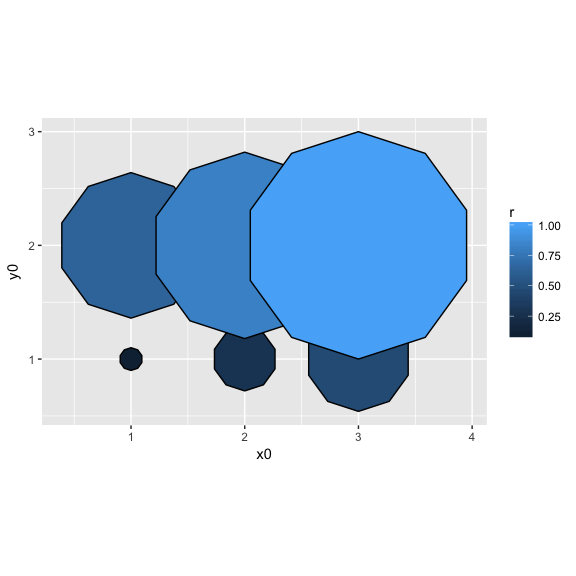## Beziers

A bezier is a smooth curve defined by its end point and one or two control points. It is well known in vector drawing software such as Adobe Illustrator, where the control points provide an intuitive way to manipulate the curve. In essence the control points define the direction and the force the curve exits the end point with - the more distant the control point is to the end point, the longer the curve travels in the direction of the control point before beginning to move towards the other end point.

There is no succinct way to describe a bezier in a single row, so all the versions use multiple rows to describe the bezier, grouped by the group aesthetic. The first row is the start point followed by one or two control points and then the end point. As bezierGrob from grid only supports quadratics beziers (2 control points) the 0-version approximates a qubic bezier by placing placing the two control points on top of each other.

``````beziers <- data.frame(
x = c(1, 2, 3, 4, 4, 6, 6),
y = c(0, 2, 0, 0, 2, 2, 0),
type = rep(c('cubic', 'quadratic'), c(3, 4)),
point = c('end', 'control', 'end', 'end', 'control', 'control', 'end')
)
help_lines <- data.frame(
x = c(1, 3, 4, 6),
xend = c(2, 2, 4, 6),
y = 0,
yend = 2
)
ggplot() + geom_segment(aes(x = x, xend = xend, y = y, yend = yend),
data = help_lines,
arrow = arrow(length = unit(c(0, 0, 0.5, 0.5), 'cm')),
colour = 'grey') +
geom_bezier(aes(x= x, y = y, group = type, linetype = type),
data = beziers) +
geom_point(aes(x = x, y = y, colour = point), data = beziers)``````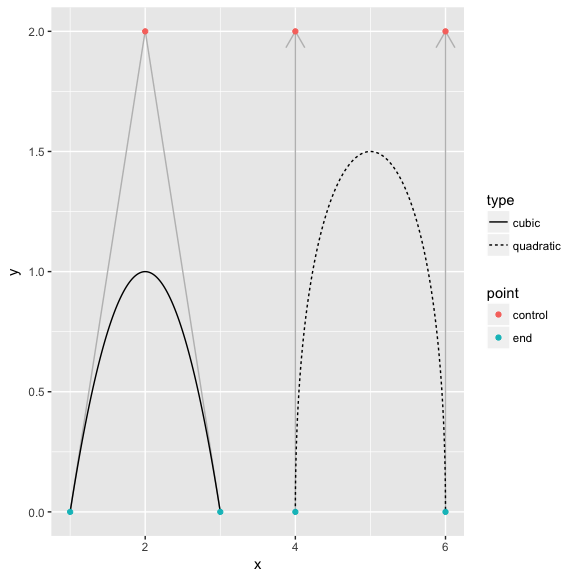## B-splines

Like beziers b-splines are smooth curves, but unlike beziers b-splines are defined by a vector of control points along which the curve will flow, without necessarily passing through any of the control points. The 0-version is impemented using xsplineGrob with `shape = 1`, which approximates a b-spline, but a slight variation is expected due to this.

``````spline <- data.frame(
x = runif(5), y = runif(5), group = 1
)
ggplot(spline) + geom_path(aes(x = x, y = y, group = group), colour = 'grey') +
geom_bspline(aes(x = x, y = y, group = group)) +
geom_point(aes(x = x, y = y))``````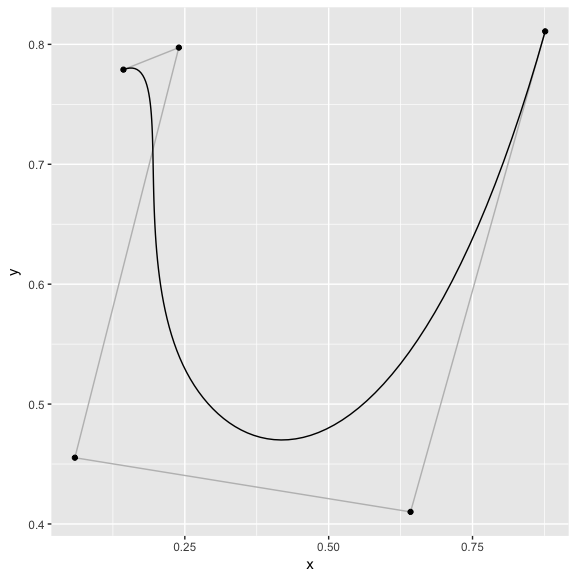## SinaPlot

`geom_sina` is inspired by the strip chart and the violin plot and operates by letting the normalized density of points restrict the jitter along the x-axis. The representation of the data as a whole remains simple, the density distribution is apparent, and the plot still provides information on how many data points are present in each class and whether outliers are driving the tails of the distribution. In this way it is possible to convey information about the mean/median of the data, its variance and the actual number of data points together with a density distribution.

``````###Sample gaussian distributions with 1, 2 and 3 modes.
df <- data.frame(
"Distribution" = c(rep("Unimodal", 500),
rep("Bimodal", 250),
rep("Trimodal", 600)),
"Value" = c(rnorm(500, 6, 1),
rnorm(200, 3, .7), rnorm(50, 7, 0.4),
rnorm(200, 2, 0.7), rnorm(300, 5.5, 0.4), rnorm(100, 8, 0.4))
)

# Reorder levels
df\$Distribution <- factor(df\$Distribution,
levels(df\$Distribution)[c(3, 1, 2)])

p <- ggplot(df, aes(Distribution, Value))
p + geom_violin(aes(fill = Distribution))``````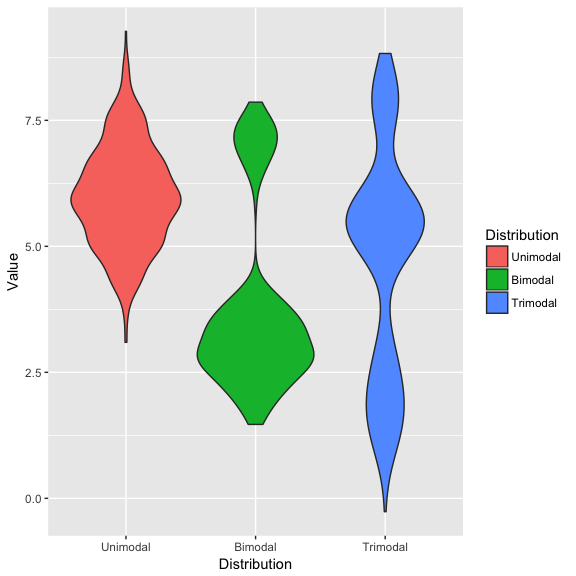``p + geom_sina(aes(color = Distribution), size = 1)``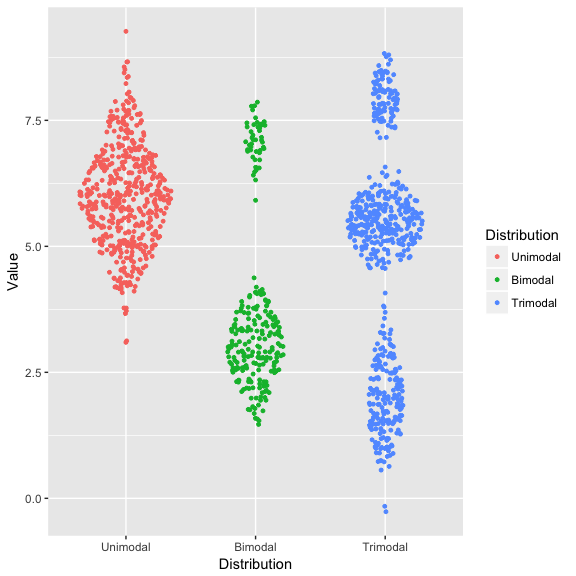# Facets

Facets has been an integral part of the success of ggplot2. With v2.2 facets extensions finally became a possibility. While the idea of facets is to create small multiples of your plots based on a set of given variables in your data, extensions are not bound by this and they can be used for any type of layout work.